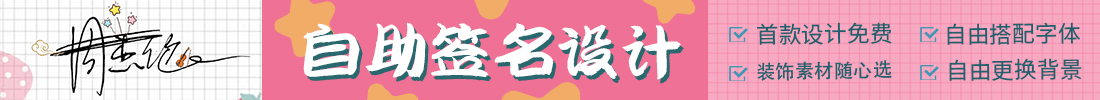• 网红签
• 免费签名
• 收费签名
• 行草签
• 连笔字
• 草书签
• 少女签
• 瘦金体
• 卡通签
• 手写体
• 立体字
• 行书签
• 星球体
• 古籍体
• 刀锋体
• 篆书签
• 楷书签
• 隶书体
• 个性签
• 签名体
• 尚乐体
• 虎汉隶
• 秋手抄
• 香珍线
• 颜黛写
• 趣卡通
• 智情体
• 钢笔行
• 无言行
• 禾文签
• 敏行签
• 心恋签
• 趣卡通
• 颜黛写
• 智情体
• 无言行
• 禾文签
• 心恋签
• 秋手抄
• 敏行签
• 香珍线
• 虎汉隶
• 钢笔行

共 1 条
• 1

# 优惠券

--暂无优惠券--
¥ {[(i.discount / 100).toFixed(2)]}
{[(i.discount / 10)]} 折

{[i.coupon_name]}

{[i.coupon_desc]}

¥ {[(i.Coupon.discount / 100).toFixed(2)]}
{[(i.Coupon.discount / 10)]} 折

{[i.Coupon.coupon_name]}

{[i.Coupon.coupon_desc]}

--暂无优惠券--